## Extract or Filter Every Nth Row

Assume that you’re in a situation where you need to filter every nth row from a list having a few values. I’m pretty sure you’d choose to do it manually, which is also a great choice when you only have a few values in a list and want to filter out every nth row.

However, if you are dealing with several values in the list and want to filter out the nth row, then executing these jobs manually would be a stupid move since there is a 90% probability that you will become tired of it and will be unable to accomplish your assignment on time.

But don’t worry, after carefully reading this post, filtering off every nth row from a list with multiple values will be a piece of cake for you.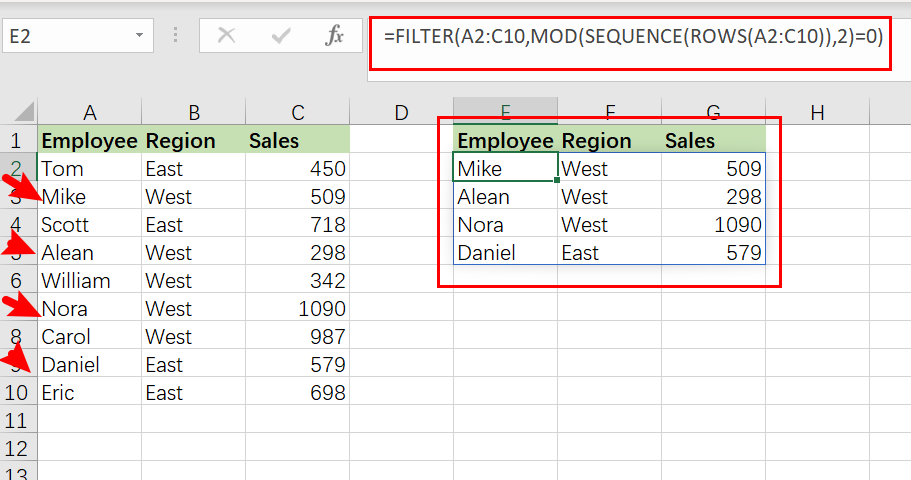So let’s go into the article and get you out of this bind.

## The formula in General

In MS Excel, use the following formula to filter every nth row:

`=FILTER(total_data,MOD(SEQUENCE(ROWS(total_data)),n)=0)`

## Explanations of Syntax

Before we get into the formula for getting the job done quickly, we need to grasp each syntax to see how each syntax helps filter out every nth row in MS Excel.

•  `Filter`: This tool helps narrow down or filter out a range of data based on user-defined criteria.
•  `Comma symbol (,)`: In Excel, this comma symbol serves as a separator, aiding in separating a list of values.
• `total_data`: is nothing more than representing each column in the list.
• `Parenthesis` (): The primary function of this Parenthesis symbol is to group and separate elements.
• `ROWS`: This refers to the nth row you want to filter out.
• ` MOD()`: The MOD function accepts two real number operands as inputs and returns the remainder of dividing the integer component of the first parameter (the dividend) by the integer part of the second argument (the divisor)
• `SEQUENCE()`: The SEQUENCE function in Excel lets you create a sequence of consecutive numbers in an array, such as 1, 2, 3, 4.

## Let’s See How This Formula Works

For example, suppose you have a task in which there is a table with candidates from two regions (i.e., West and East ) and which are assigned to a particular sales number; now you want to filter out every second row, which is the sequence which we would write in the formula; now let’s analyze how to write the formula and how this formula would do it.

To filter every second row, we would use the following formula based on the supplied list:

`=FILTER(A2:C10,MOD(SEQUENCE(ROWS(A2:C10)),2)=0)`To filter and extract every nth row, use a formula that combines the FILTER function with `MOD`, `ROW`, and `SEQUENCE`. Where data refers to the designated range A2:C10. With n hardcoded as `2`, the FILTER function retrieves every third row in the data.

The FILTER function filters and extracts data based on logical criteria. This example aims to extract every second record from the supplied data, although there is no row number information in the data range A2:C10.

The first stage, working from the inside out, is establishing a row number set. This is accomplished using the SEQUENCE function as follows:

`=SEQUENCE(ROWS(A2:C10))`

The ROW function returns the number of rows in the specified range of data. SEQUENCE provides an array of 9 integers in sequence based on the number of rows:

`{1;2;3;4;5;6;7;8;9}`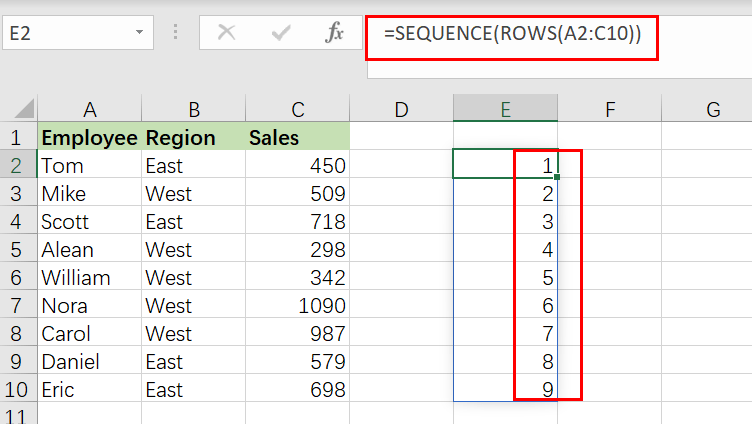This array is sent straight to the MOD function as the number parameter, with the divisor hardcoded as 2. MOD is configured to check if row numbers are divisible by 2 with a remainder of zero.

`=MOD(SEQUENCE(ROWS(A2:C10)),2)=0 /// is it divisible by 2?`

MOD produces an array of TRUE and FALSE values, as shown below:

`{FALSE;TRUE;FALSE;TRUE;FALSE;TRUE;FALSE;TRUE;FALSE}`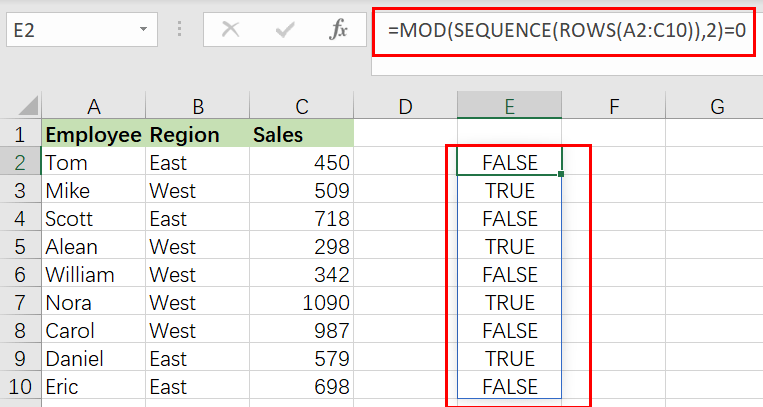Take note that TRUE values correlate to every second row in the data range A2:C10. This array is passed straight to the FILTER function as the include parameter. As a final result, FILTER returns every second row of data.

### Related Functions

• Excel Filter function
The Excel  FILTER function extracts matched records from a collection of data using one or more logical checks. The include argument specifies logical tests, which might encompass a wide variety of formula conditions.==FILTER(array,include,[if empty])…
• Excel MOD function
the Excel MOD function returns the remainder of two numbers after division. So you can use the MOD function to get the remainder after a number is divided by a divisor in Excel. The syntax of the MOD function is as below:=MOD (number, divisor)….
• Excel ROWS function
The Excel ROWS function returns the number of rows in a cell reference.The syntax of the ROWS function is as below:= ROWS(array)…

## How to Convert Horizontal List of Data into Vertical in Excel

This post will guide you how to transpose horizontal list to vertical list in your current worksheet in Excel. How do I convert horizontal rows to vertical columns with a formula in Excel 2013/2016.

Assuming that you have a list of data (A1:E1) in a Microsoft Excel worksheet, and you want to realize that the data you entered in rows makes better sense in columns or vice versa. You need to transpose your rows and columns from horizontal list to vertical list. This post will show you two methods to change the horizontal row style to vertical style.

## Convert Horizontal List to Vertical with a Formula

If you want to convert horizontal list to a vertical list, you can create a formula based on the INDEX function in combination with ROWS function. Like this:

`=INDEX(A\$1:E\$1, ROWS(F\$1:F1))`

Then enter this formula into a blank cell such as: Cell F1, and then press Enter key on your keyboard, and the drag the AutoFill Handle down to other cells, until you get a #REF! error message.

Note: Cell A1:E1 is your horizontal list, and F1 is the first cell that you want to place vertical list. It is also the cell that you want to apply for the formula.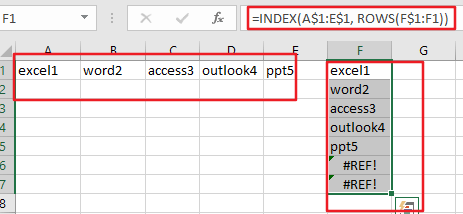You would see that the horizontal list has been converted to vertical list.

## Convert Horizontal List to Vertical using Paste Special Feature

You can also use Paste Special function to achieve the same result of transposing horizontal row data into vertical column style in Excel. Just do the following steps:

Step1: select cells that you want to convert, such as: A1:E1.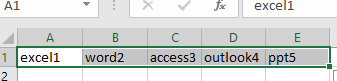Step2: right click on the selected cells, and select Copy option from the popup context menu.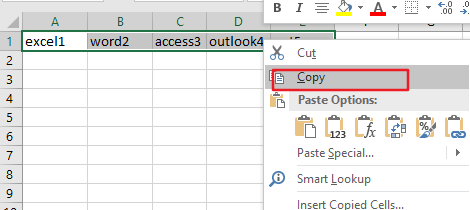Step3: select a blank cell that you want to place vertical list, such as: Cell F1. And right click on it , and choose Paste Special from the popup menu list. Then the Paste Special dialog will open.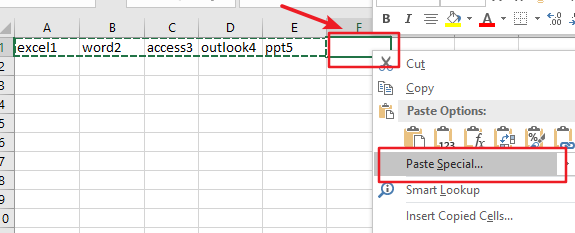Step4: check the Transpose box in the Paste Special dialog box. Then click Ok button.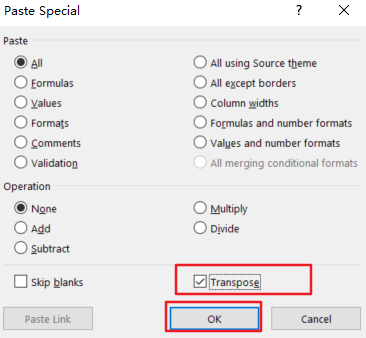Step5: you would now see your horizontal data be transposed to vertical style.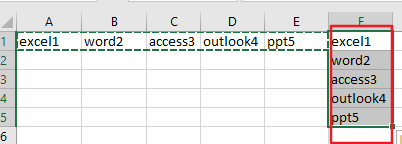### Related Functions

• Excel INDEX function
The Excel INDEX function returns a value from a table based on the index (row number and column number)The INDEX function is a build-in function in Microsoft Excel and it is categorized as a Lookup and Reference Function.The syntax of the INDEX function is as below:= INDEX (array, row_num,[column_num])…
• Excel ROWS function
The Excel ROWS function returns the number of rows in a cell reference.The syntax of the ROWS function is as below:= ROWS(array)…

## Copy and Paste Only Non-blank Cells

This post will guide you how to copy and paste only non-blank cells in Excel 2013/2016. How do I copy only non blank cells with VBA code in Excel. Or how to get non-blank cells from a range of cells with a formula in Excel.

This post will introduce four methods to copy and paste only non-blank cells in a selected range in Excel.

## Method1: Copy non-blank cells with Filter feature

If you want only copy non-blank cells in a range in Excel, you need to select the non-blank cells firstly, then press Ctrl +C keys to copy the selected cells. So how to only select all non-blank cells in the selected range in your worksheet. Just do the following steps:

#1 select the range of cells that you want to filter all non-blank cells.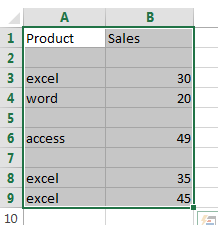#2 go to DATA tab, click Filter command under Sort & Filter group.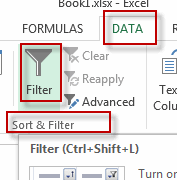#3 the drop-down button should appear in the first cell of your range. Click on this drop down button. de-select the (Blanks) option and then click on the OK button.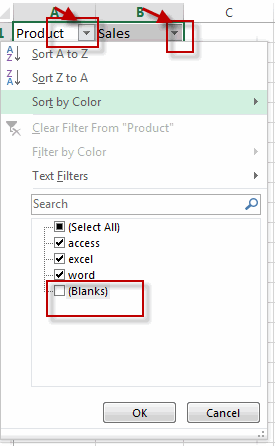#4 you will see that only non-blank cells are displayed in the selected range.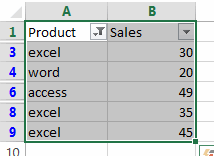#5 then you can select all non-blank cells in the current range and press Ctrl + C shortcuts in your keyboard, and then press Ctrl + V keys to paste the selected cells into a destination cell.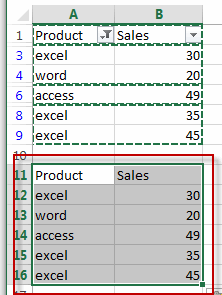## Method2: Copy non-blank Cells with Go To Special Feature

You can also use the Go To Special feature to select all non-blank cells in the selected range of cells. Do the following steps:

#1 select the range of cells that you want to filter all non-blank cells.#2 go to HOME tab, click Find & Select command under Editing group. And select Go To Special from the popup menu list. The Go To Special window will open.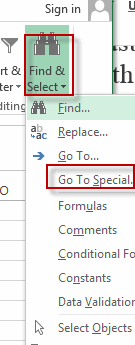#3 select Constants radio button in the Select section under Go To Special dialog. Then click OK button.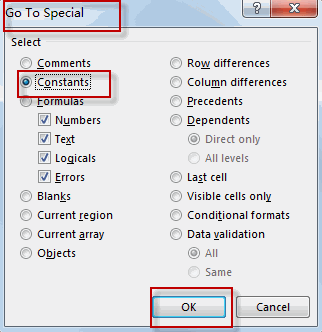#4 all non-blank cells in the selected range are highlighted.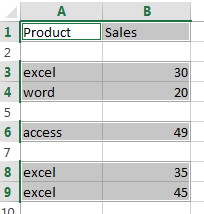#5 you can press Ctrl + C shortcuts to copy cells have been highlighted, and then press Ctrl + V keys to paste the selected cells into a destination cell.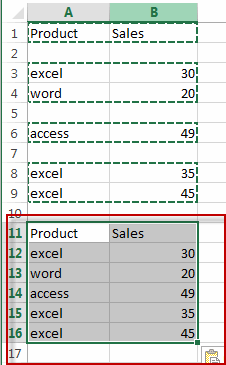## Method3: Copy non-blank Cells with VBA Macro

You can also use an Excel array formula based on the LOOKUP function, the CHOOSE function, the INDEX function, the SMALL function, the IF function, the ROW function and the ROWS function to extract all non-blank cells from a cell. Like this:

`=LOOKUP("xxxxx",CHOOSE({1,2},"",INDEX(A:A,SMALL(IF(\$A\$1:\$A\$9<>"",ROW(\$A\$1:\$A\$9)),ROWS(\$B\$1:B1)))))`

Type this formula into cell B1, and then press Ctrl +Shift +Enter shortcuts to change this formula as array formula. And then drag the AutoFill Handle from Cell B1 to B9.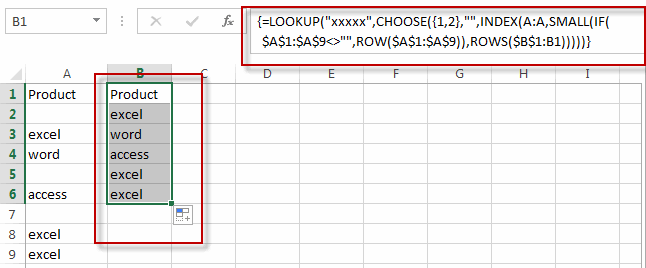All non-blank cells from the range A1:A9 are extracted in the range B1:B9.

## Method4: Copy non-blank Cells with Formula

You can also write an Excel VBA macro to copy and paste all non-blank cells, just do the following steps:

#1 select the range of cells that you want to filter all non-blank cells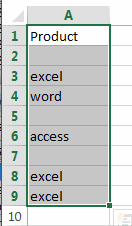#2 open your excel workbook and then click on “Visual Basic” command under DEVELOPER Tab, or just press “ALT+F11” shortcut.#3 then the “Visual Basic Editor” window will appear.

#4 click “Insert” ->”Module” to create a new module.#5 paste the below VBA code into the code window. Then clicking “Save” button.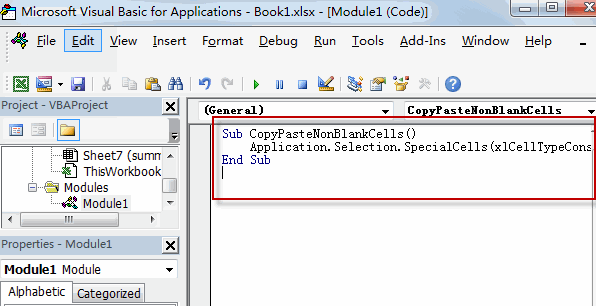```Sub CopyPasteNonBlankCells()
Application.Selection.SpecialCells(xlCellTypeConstants).Copy Destination:=Range("B1")
End Sub```

#6 back to the current worksheet, then run the above excel macro. Click Run button.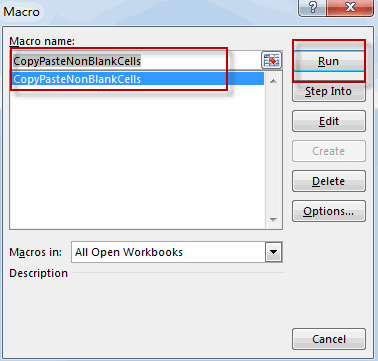#7 let’s see the result.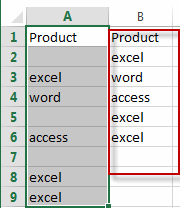### Related Functions

• Excel LOOKUP function
The Excel LOOKUP function will search a value in a vector or array.The LOOKUP function is a build-in function in Microsoft Excel and it is categorized as a Lookup and Reference Function.The syntax of the LOOKUP function is as below:= LOOKUP (lookup_value, lookup_vector, [result_vector])…
• Excel Choose Function
The Excel CHOOSE function returns a value from a list of values. The CHOOSE function is a build-in function in Microsoft Excel and it is categorized as a Lookup and Reference Function.The syntax of the CHOOSE function is as below:=CHOOSE (index_num, value1,[value2],…)…
• Excel INDEX function
The Excel INDEX function returns a value from a table based on the index (row number and column number)The INDEX function is a build-in function in Microsoft Excel and it is categorized as a Lookup and Reference Function.The syntax of the INDEX function is as below:= INDEX (array, row_num,[column_num])…
• Excel ROWS function
The Excel ROWS function returns the number of rows in a cell reference.The syntax of the ROWS function is as below:= ROWS(array)…
• Excel SMALL function
The Excel SMALL function returns the smallest numeric value from the numbers that you provided. Or returns the smallest value in the array.The syntax of the SMALL function is as below:=SMALL(array,nth) …
• Excel ROW function
The Excel ROW function returns the row number of a cell reference.The ROW function is a build-in function in Microsoft Excel and it is categorized as a Lookup and Reference Function.The syntax of the ROW function is as below:= ROW ([reference])….
• Excel IF function
The Excel IF function perform a logical test to return one value if the condition is TRUE and return another value if the condition is FALSE. The IF function is a build-in function in Microsoft Excel and it is categorized as a Logical Function.The syntax of the IF function is as below:= IF (condition, [true_value], [false_value])….

## Split Data in One Column to Multiple Columns

This post will guide you how to split data in a single column into multiple columns with a formula in Excel. How do I transpose data from one column to multiple columns in Excel. How can I make one long column into multiple columns in Excel.

## Split Data in One Column to Multiple Columns

Assuming that you have 5 sets of product’s list in one column, and you would like to convert each set in its own column or split each sets into its own staked columns. How to achieve it.

If you want to split each sets in one column from row to row in stacked columns, and you need to create a formula based on the OFFSET function, the COLUMNS function, and the ROWS function.

Just like this:

`=OFFSET(\$A\$1,COLUMNS(\$A1:A1)-1+(ROWS(\$1:1)-1)*2,0)`

Type this formula into a blank cell C1, and press Enter key in your keyboard, and then drag the AutoFill Handle from C1 to D1. Select the Cell C1 and D1, then drag the AutoFill Handle in Cell D1 from D1 to D5.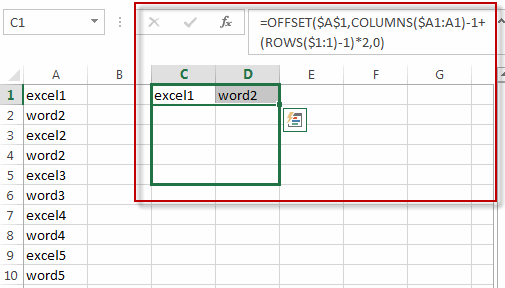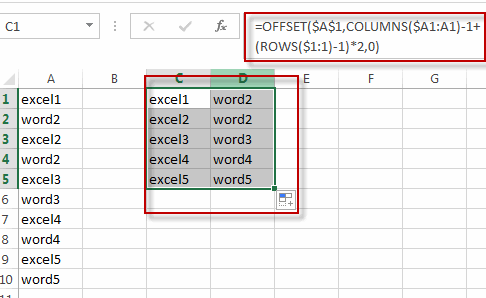If you want to split each sets in one column from one column into another column, you just need to use the following formula based on the INDEX function, the ROW function and the COLUMNS function. Like this:

`=INDEX(\$A\$1:\$A\$10,ROW(C1)+(2*(COLUMNS(\$C\$1:C\$1)-1)))`

Type this formula into the formula box of the Cell C1, and press Enter key in your keyboard, and drag the AutoFill Handle from C1 to C2. Select the Cell C1 and C2, and then drag the AutoFill handle in Cell C2 from C2 to G2.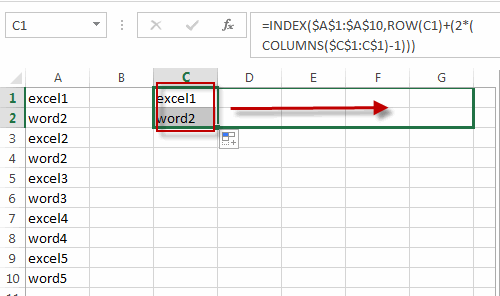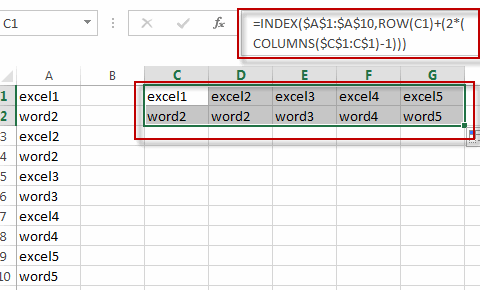### Related Functions

• Excel INDEX function
The Excel INDEX function returns a value from a table based on the index (row number and column number)The INDEX function is a build-in function in Microsoft Excel and it is categorized as a Lookup and Reference Function.The syntax of the INDEX function is as below:= INDEX (array, row_num,[column_num])…
• Excel ROW function
The Excel ROW function returns the row number of a cell reference.The ROW function is a build-in function in Microsoft Excel and it is categorized as a Lookup and Reference Function.The syntax of the ROW function is as below:= ROW ([reference])….
• Excel ROWS function
The Excel ROWS function returns the number of rows in a cell reference.The ROWS function is a build-in function in Microsoft Excel and it is categorized as a Lookup and Reference Function.The syntax of the ROWS function is as below:= ROWS(array)….
• Excel COLUMNS function
The Excel COLUMNS function returns the number of columns in an Array or a reference.The syntax of the COLUMNS function is as below:=COLUMNS (array)….

## List all Worksheet Names

This post will guide you how to get a list of all worksheet names in an excel workbook. How do I List the Sheet names with Formula in Excel. How to generate a list of all sheet tab names using Excel VBA Code.

Assuming that you have a workbook that has hundreds of worksheets and you want to get a list of all the worksheet names in the current workbook. And the below will introduce 3 methods with you.

## Get All Worksheet Names Manually

If there are only few worksheets in your workbook, and you can get a list of all worksheet tab names by manually. Let’s see the below steps:

#2 double click on the sheet’s name in the sheet tab. Press Ctrl + C shortcuts in your keyboard to copy the selected sheet.

#3 create a notepad file, and then press Ctrl +V to paste the sheet name.

#4 follow the above steps 2-3 to copy&paste all worksheet names into notepad file.

## Get All Worksheet Names with Formula

You can also use a formula to get a list of all worksheet names with a formula. You can create a formula based on the LOOKUP function, the CHOOSE function, the INDEX function, the MID function, the FIND function and the ROWS function. Just do the following steps:

#1 go to FORMULAS tab, click Name Manager command under Defined Names group. The Name Manager dialog will open.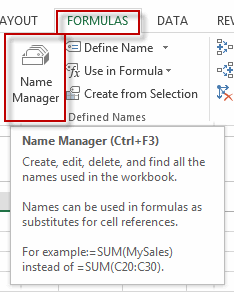#2 click New… button to create a define name, type Sheets in the Name text box, and type the formula into the Refers to text box.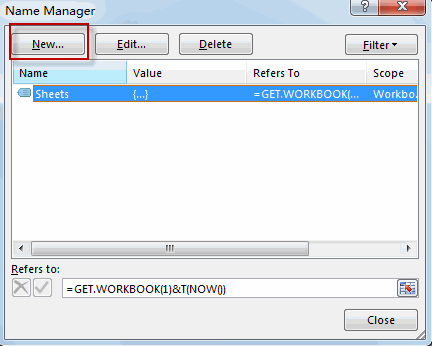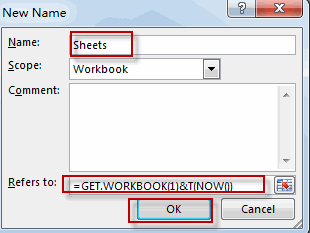`=GET.WORKBOOK(1)&T(NOW())`

#3 Type the following formula into a blank cell and press Enter key in your keyboard, and then drag the autofill handle over others cells to get the rest sheet names.

`=LOOKUP("xxxxx",CHOOSE({1,2},"",INDEX(MID(Sheets,FIND("]",Sheets)+1,255),ROWS(A\$1:A1))))`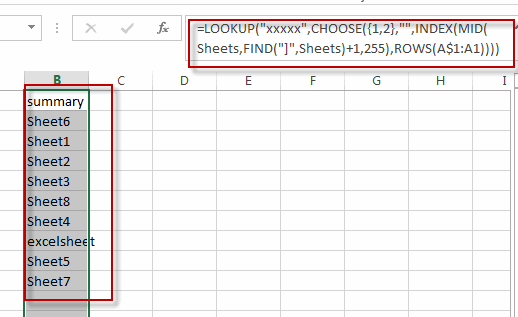You will see that all sheet names have been listed in the cells.

## Get All Sheet Names with Excel VBA Macro

You can also use an Excel VBA Macro to quickly get a list of all worksheet tab names in your workbook. Just do the following steps:

#1 open your excel workbook and then click on “Visual Basic” command under DEVELOPER Tab, or just press “ALT+F11” shortcut.#2 then the “Visual Basic Editor” window will appear.

#3 click “Insert” ->”Module” to create a new module#4 paste the below VBA code into the code window. Then clicking “Save” button.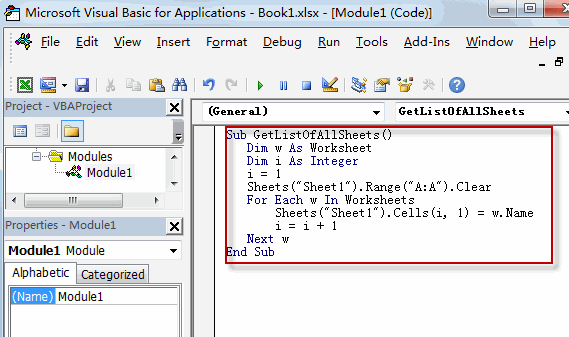```Sub GetListOfAllSheets()
Dim w As Worksheet
Dim i As Integer
i = 1
Sheets("Sheet1").Range("A:A").Clear
For Each w In Worksheets
Sheets("Sheet1").Cells(i, 1) = w.Name
i = i + 1
Next w
End Sub```

#5 back to the current worksheet, then run the above excel macro. Click Run button.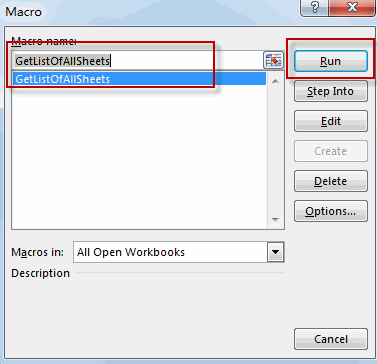#6 Let’s see the result.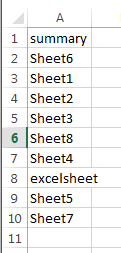### Related Functions

• Excel MID function
The Excel MID function returns a substring from a text string at the position that you specify.The syntax of the MID function is as below:= MID (text, start_num, num_chars)…
• Excel LOOKUP function
The Excel LOOKUP function will search a value in a vector or array.The LOOKUP function is a build-in function in Microsoft Excel and it is categorized as a Lookup and Reference Function.The syntax of the LOOKUP function is as below:= LOOKUP (lookup_value, lookup_vector, [result_vector])…
• Excel Choose Function
The Excel CHOOSE function returns a value from a list of values. The CHOOSE function is a build-in function in Microsoft Excel and it is categorized as a Lookup and Reference Function.The syntax of the CHOOSE function is as below:=CHOOSE (index_num, value1,[value2],…)…
• Excel INDEX function
The Excel INDEX function returns a value from a table based on the index (row number and column number)The INDEX function is a build-in function in Microsoft Excel and it is categorized as a Lookup and Reference Function.The syntax of the INDEX function is as below:= INDEX (array, row_num,[column_num])…
• Excel ROWS function
The Excel ROWS function returns the number of rows in a cell reference.The syntax of the ROWS function is as below:= ROWS(array)…
• Excel FIND function
The Excel FIND function returns the position of the first text string (sub string) within another text string.The syntax of the FIND function is as below:= FIND(find_text, within_text,[start_num])

## Randomly Select Cells

This post will guide you how to randomly select cells from a list or table in Excel. How to get random value form a range of cells in an Excel Spreadsheet.

Assuming that you have a list of data (B1:B7) in a worksheet, and you want to get 3 random cells or values from those data, how to achieve it. This post will introduce two method to get random value from a range of cells. Randomly Select Cells with Formula. Or how to use an Excel User Defined Function to select cells randomly.

## Randomly Select Cells with a Formula

If you want to randomly select cells from a range of cells, you can use a formula based on the INDEX function, the RANDBETWEEN function and the Rows function. Like this:

`=INDEX(\$B\$1:\$B:\$7,RANDBETWEEN(1,ROWS(\$B\$1:\$B:\$7)),1)`

Type this formula into a blank cell C1, and press Enter key in your keyboard, and then drag the AutoFill Handle over other two cells to apply this formula to get 3 random cells.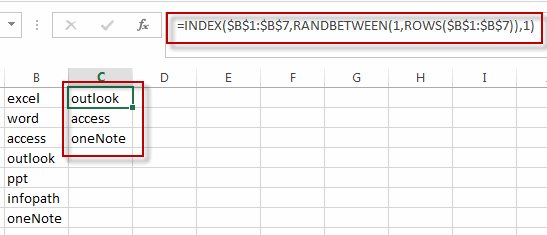## Randomly Select Cells with a User Defined Function

You can also write an User Defined Function with VBA code to quickly select random cells from a range of cells in Excel. Just do the following steps:

#1 open your excel workbook and then click on “Visual Basic” command under DEVELOPER Tab, or just press “ALT+F11” shortcut.#2 then the “Visual Basic Editor” window will appear.

#3 click “Insert” ->”Module” to create a new module.#4 paste the below VBA code into the code window. Then clicking “Save” button.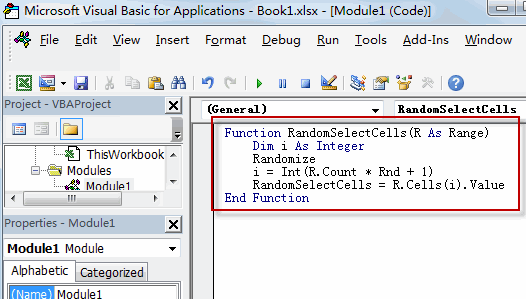```Function RandomSelectCells(R As Range)
Dim i As Integer
Randomize
i = Int(R.Count * Rnd + 1)
RandomSelectCells = R.Cells(i).Value
End Function```

#5 back to the current worksheet, try to enter the below formula in Cell C1.

`=RandomSelectCells(\$B\$1:\$B\$7)`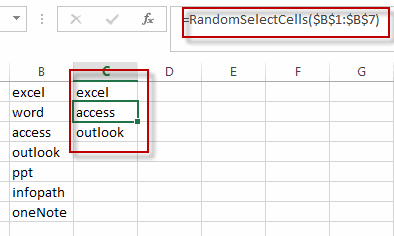### Related Functions

• Excel INDEX function
The Excel INDEX function returns a value from a table based on the index (row number and column number)The INDEX function is a build-in function in Microsoft Excel and it is categorized as a Lookup and Reference Function.The syntax of the INDEX function is as below:= INDEX (array, row_num,[column_num])…
• Excel RANDBETWEEN Function
The Excel RANDBETWEEN function returns a random integer number that is between the numbers you specify.The syntax of the RANDBETWEEN function is as below:= RANDBETWEEN (bottom,top)….
• Excel ROWS function
The Excel ROWS function returns the number of rows in a cell reference.The syntax of the ROWS function is as below:= ROWS(array)…

## Flip a Column of Data Vertically

This post will guide you how to flip a column of data vertically preserving the original formatting and formulas in Excel. How do I reverse a column of data order in a column arranged alphabetically or from largest to smallest. How to use the Excel Sort feature to reverse the data order? Or how to use a VBA macro code to flip a column of data vertically in Excel.

If you want to flip or reverse a column of data that is already in numeric or alphabetical order, and you can easily to flip it with Sort feature. And if the column data is not sorted and you can try to create a helper column to achieve the result.

## Flip a Column of Data with Sort Feature

#1 select the column that you want to flip, right click on it, and select Insert to add helper column. Choose Entire column radio button. Then insert 1,2,3,4,…in helper column.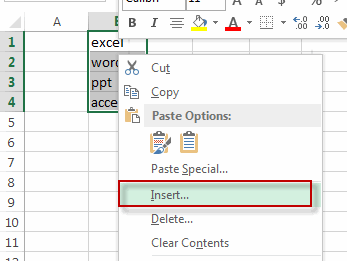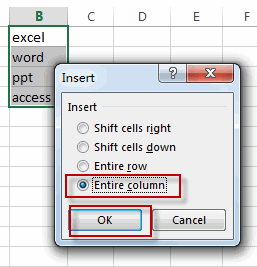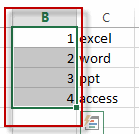#2 select your helper column, then go to the Data tab, click the Sort Largest to Smallest command under Sort&Filter group.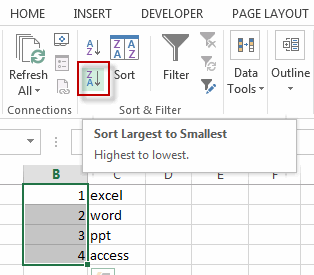#3 you will see that the numbers in help column will be sorted and the data of column also be reversed. Now you can delete the help column. Or you can also hide the help column in case you want to restore the data of column.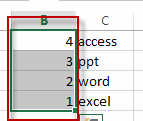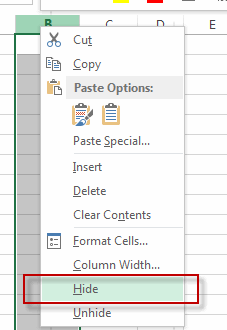#4 let’s see the result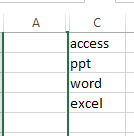## Flip a Column of Data Vertically with Function

You can also create an excel formula based on the INDEX function and the ROWS function to flip a column of data vertically. Type the following formula in the formula box of cell C1, then press enter key.

`=INDEX(\$B\$1:\$B\$4,ROWS(B1:\$B\$4))`

Let’s see the result as below: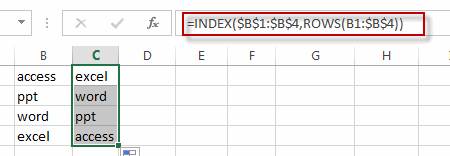## Flip a Column of Data Vertically with VBA Macro

You can also write an Excel VBA Macro to flip a column of data vertically quickly. Just do the following steps:

#1 click on “Visual Basic” command under DEVELOPER Tab.#2 then the “Visual Basic Editor” window will appear.

#3 click “Insert” ->”Module” to create a new module.#4 paste the below VBA code into the code window. Then clicking “Save” button.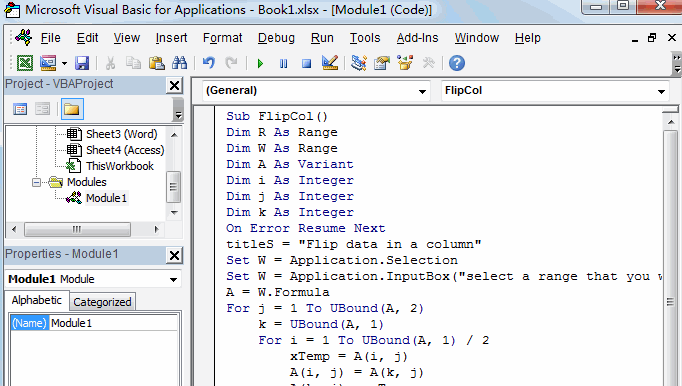```Sub FlipCol()
Dim R As Range
Dim W As Range
Dim A As Variant
Dim i As Integer
Dim j As Integer
Dim k As Integer
On Error Resume Next
titleS = "Flip data in a column"
Set W = Application.Selection
Set W = Application.InputBox("select a range that you want to reverse", titleS, W.Address, Type:=8)
A = W.Formula
For j = 1 To UBound(A, 2)
k = UBound(A, 1)
For i = 1 To UBound(A, 1) / 2
xTemp = A(i, j)
A(i, j) = A(k, j)
A(k, j) = xTemp
k = k - 1
Next
Next
W.Formula = A
End Sub```

#5 back to the current worksheet, then run the above excel macro. Click Run button.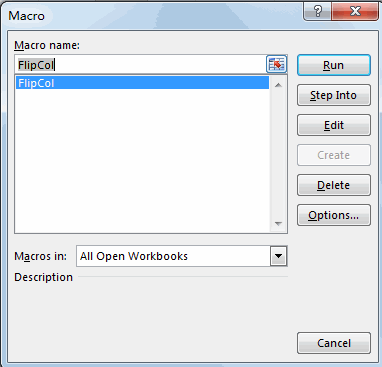#6 select a range that you want to flip.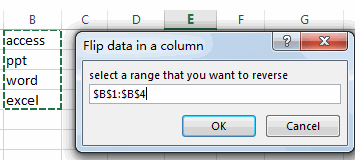#7 click OK button, then check the result.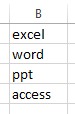### Related Functions

• Excel INDEX function
The Excel INDEX function returns a value from a table based on the index (row number and column number)The INDEX function is a build-in function in Microsoft Excel and it is categorized as a Lookup and Reference Function.The syntax of the INDEX function is as below:= INDEX (array, row_num,[column_num])…
• Excel ROWS function
The Excel ROWS function returns the number of rows in a cell reference.The syntax of the ROWS function is as below:= ROWS(array)…

## How to Reverse a List or Range

This post will guide you how to reverse a list of values or a range of values in excel. How to reverse the order of a column data or a list of items using excel formula. How to reverse a range with sort command. How to reverse a list or column value with VBA.

## Reverse a List or Range with Formula

If you want to reverse a list or range, you can use a combination of the INDEX function, the COUNTA function, the ROW function or ROWS function to create a new formula.

For example, to reverse the range A2:A5, you can use the following formula:

`=INDEX(\$A\$2:\$A\$5,COUNTA(\$A\$2:\$A\$5)-ROWS(\$C\$2:C2)+1)`

Let’s see how the above formulas works:

= COUNTA(\$A\$2:\$A\$5)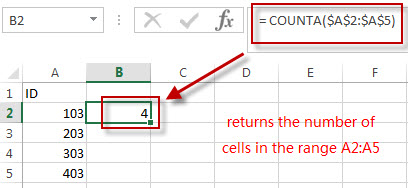The COUNTA function returns the number of cells in the range A2:A5.  The above COUNTA formula used the absolute reference as its arguments, so it won’t be changed when dragging Fill handle to other single cell.

=ROWS(\$C\$2:C2)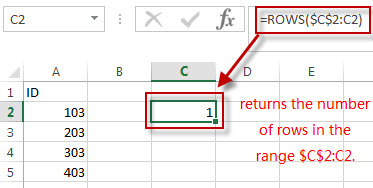The ROWS function returns the number of rows in the range \$C\$2:C2. The first Cell is an absolute reference and the second Cell is a relative reference in the argument of the ROWS function. So the second Cell reference can be changed when dragging the Fill handle.

= COUNTA(\$A\$2:\$A\$5)-ROWS(\$C\$2:C2)+1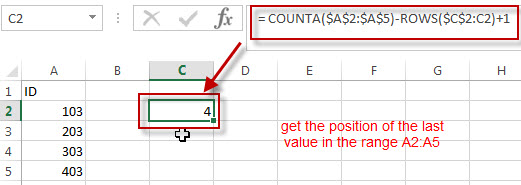The number of Cells in the range (\$A\$2:\$A\$5 subtracted the number of rows in the range \$C\$2:C2, then add 1, it returns the position of the last value in the range A2:A5. And it returns 4.

=INDEX(\$A\$2:\$A\$5,COUNTA(\$A\$2:\$A\$5)-ROWS(\$C\$2:C2)+1)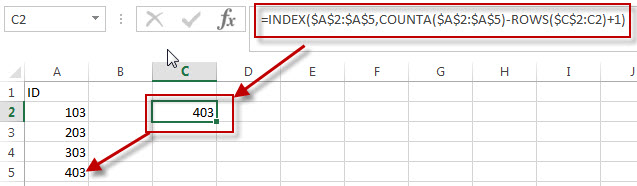We can use the INDEX function to extract the value based on the position result returned by the above COUNTA-ROWS formula.  So it returns value 403.

Next, we just need to drag the Fill Handler in Cell C1 to other single cells, such as: C2,C3,C4…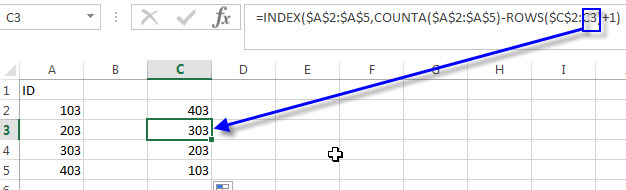You can also use the below two excel formula to reverse a list or a range:

=INDEX(\$A\$2:\$A\$5,ROWS(C2:C\$5))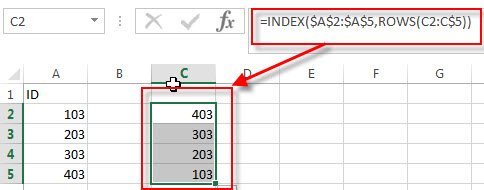=INDEX(\$A\$2:\$A\$5, COUNTA(\$A\$2:\$A\$5) + ROW(\$A\$2:\$A\$5)-ROW(),1)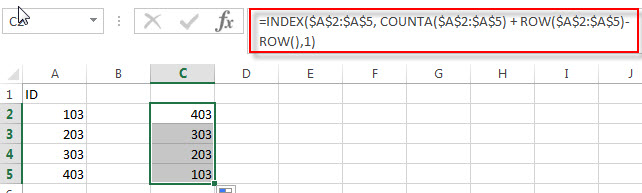## Reverse a List or Range with Sort command

You can also use the Sort command to reverse a list or a range in excel, for example, if you want to reverse the values in the range A2:A5, just refer to the following steps:

1# enter the value 1 into the cell B2, and then fill cells to B5 with a series by using the fill handle.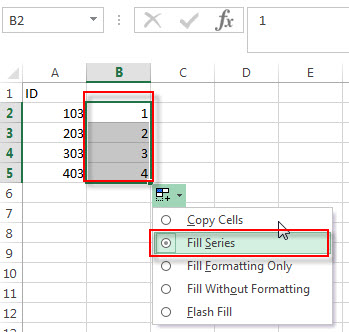2# click any cell in the range B2:B5

3# click “Sort Z to A”command under Data tab.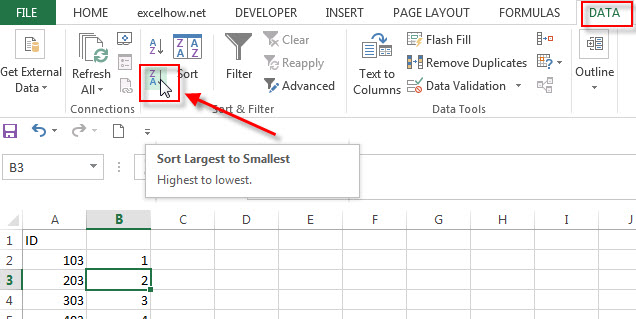4# delete the Column B, you will see that the range A2:A5 is reversed.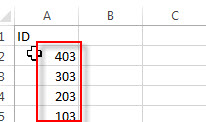## Reverse a List or Range with VBA

You can follow the below steps to create a new excel macro to reverse a list or range in Excel VBA:

1# click on “Visual Basic” command under DEVELOPER Tab.2# then the “Visual Basic Editor” window will appear.

3#click “Insert” ->”Module” to create a new module.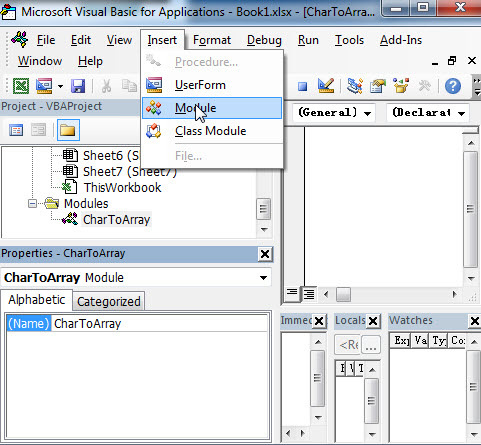4# paste the below VBA code into the code window. Then clicking “Save” button.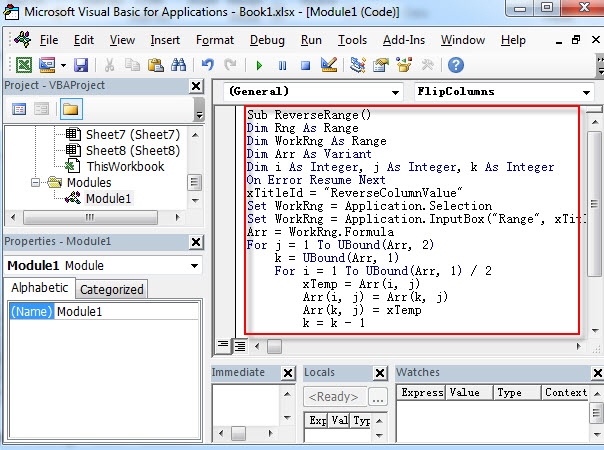```Sub ReverseRange()
Dim Rng As Range
Dim WorkRng As Range
Dim Arr As Variant
Dim i As Integer, j As Integer, k As Integer
On Error Resume Next
xTitleId = "ReverseColumnValue"
Set WorkRng = Application.Selection
Set WorkRng = Application.InputBox("Range", xTitleId, WorkRng.Address, Type:=8)
Arr = WorkRng.Formula
For j = 1 To UBound(Arr, 2)
k = UBound(Arr, 1)
For i = 1 To UBound(Arr, 1) / 2
xTemp = Arr(i, j)
Arr(i, j) = Arr(k, j)
Arr(k, j) = xTemp
k = k - 1
Next
Next
WorkRng.Formula = Arr
End Sub```

5# back to the current workbook, then click Macros button under DEVELOPER tab, or Press F5 key to run the above macro.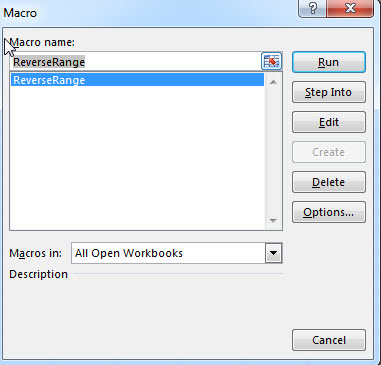6# click “RUN” button, then input the range value A2:A5 that you want to reverse.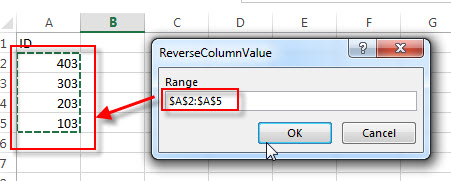7# let’s see that last result.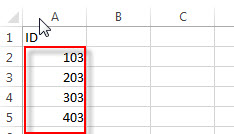### Related Formulas

• Find the Relative Position in a Range or Table
If you want to know the relative row position for all rows in an Excel Range (B3:D6), you can use a excel Array formula as follows:=ROW(B3:D6)- ROW(B3) + 1. You can also use another excel array formula to get the same result as follows:=ROW(B3:D6)-ROW(INDEX(B3:D6,1,1))+1…
• Lookup the Next Largest Value
If you want to get the next largest value in another column, you can use a combination of the INDEX function and the MATCH function to create an excel formula.you can use the following formula:=INDEX(A2:A5,MATCH(200,A2:A5)+1)…

### Related Functions

• Excel INDEX function
The Excel INDEX function returns a value from a table based on the index (row number and column number)The INDEX function is a build-in function in Microsoft Excel and it is categorized as a Lookup and Reference Function.The syntax of the INDEX function is as below:= INDEX (array, row_num,[column_num])…
• Excel ROW function
The Excel ROW function returns the row number of a cell reference.The ROW function is a build-in function in Microsoft Excel and it is categorized as a Lookup and Reference Function.The syntax of the ROW function is as below:= ROW ([reference])….
• Excel COUNTA function
The Excel COUNTA function counts the number of cells that are not empty in a range. And it returns the number of non-blank cells within a range or value. The syntax of the COUNTA function is as below:= COUNTA(value1, [value2],…)
• Excel ROWS function
The Excel ROWS function returns the number of rows in a cell reference.The ROWS function is a build-in function in Microsoft Excel and it is categorized as a Lookup and Reference Function.The syntax of the ROWS function is as below:= ROWS(array)…

## How to Extract multiple match Values into different Columns or Rows

This post explains that how to extract multiple match values from a range, then put the values into the different columns in excel. And how to extract multiple matches into the different rows.

In the previous post, we talked that how to get the position of the nth occurrence of a value in a column, and this pose will refer to it to extract all matched values firstly, then put all returned values into the different columns.

## Extract multiple match values into separate columns

If you want to fetch all matches from a range then put it into cells in different columns, you can use a combination with the INDEX function, the SMALL function, the IF function,  the ROW function and the COLUMNS function to create a new excel formula.

For example, if you want to get all member names belong to “excel” team in the range B3:D7, then separate it into the different columns, such as: E2, F2…you can use the following formula:

`=IFERROR(INDEX(\$D\$3:\$D\$7,SMALL(IF(\$C\$3:\$C\$7="excel",ROW(\$C\$3:\$C\$7)-ROW(INDEX(\$C\$3:\$C\$7,1,1))+1),COLUMNS(\$E\$2:E2))),"")`

Let’s see how the above formula works:

= ROW(\$C\$3:\$C\$7)-ROW(INDEX(\$C\$3:\$C\$7,1,1))+1

The ROW function returns the relative position of all rows in the range C3:C7.  The returned result is another array.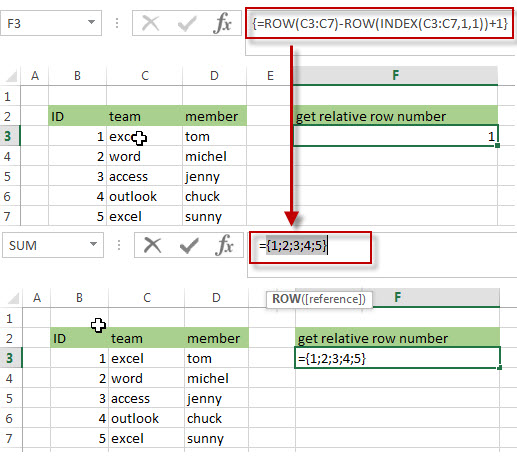= IF(\$C\$3:\$C\$7=”excel”,ROW(\$C\$3:\$C\$7)-ROW(INDEX(\$C\$3:\$C\$7,1,1))+1)

The IF function will check if each value in range C3:C7 is equal to “excel”, if TRUE, returns its relative position, otherwise, returns FALSE. As this formula is an array formula, so it must be returned an array result.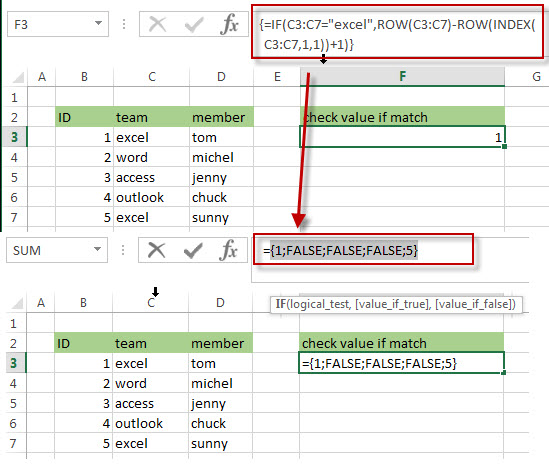=COLUMNS(\$E\$2:E2)

The COLUMNS function returns a numeric values, it will be used by the SMALL function as its nth argument to indicate the position of the number that you want to return.

=SMALL(IF(\$C\$3:\$C\$7=”excel”,ROW(\$C\$3:\$C\$7)-ROW(INDEX(\$C\$3:\$C\$7,1,1))+1),COLUMNS(\$E\$2:E2))

The SMALL function returns the position of the nth smallest values from the numbers in an array result returned by the above IF function. The nth argument is specified by the result returned by the COLUMNS function.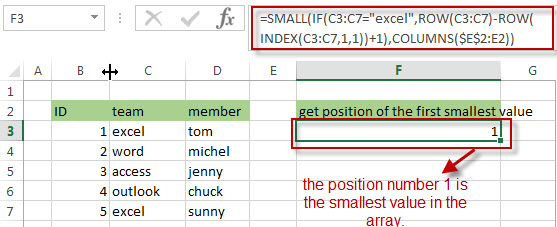=INDEX(\$D\$3:\$D\$7,SMALL(IF(\$C\$3:\$C\$7=”excel”,ROW(\$C\$3:\$C\$7)-ROW(INDEX(\$C\$3:\$C\$7,1,1))+1),COLUMNS(\$E\$2:E2)))

The INDEX function returns a value at a position returned by the above SMALL function in the range D3:D7, so it should be the first matches of the member name that belong to the excel team in the range B3:D7.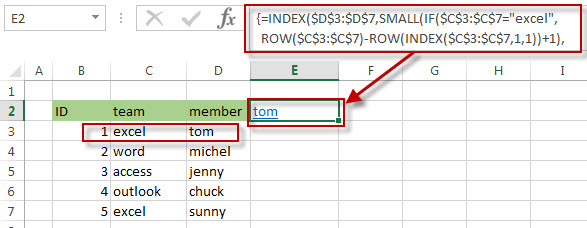And if you want to get the second matches into Cell F2, you just need to drag the fill hander in the Cell E2 to F2, then the second matches of the member name will be fetched in the Cell F2.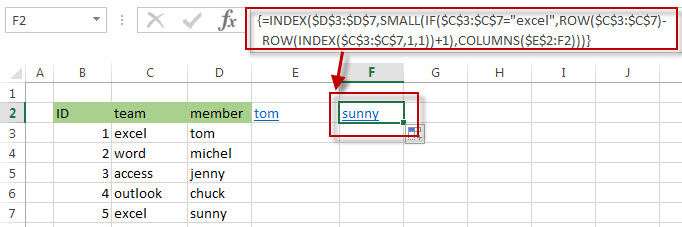=IFERROR()

The IFERROR function will check if the result returned by the SMALL function is a #NUM error, then returns an empty string.

## Extract multiple match values into separate rows

If you want to extract multiple matches into the separate rows in excel, just need to use ROWS instead of the COLUMNS function in the above formula as follows:

`=IFERROR(INDEX(\$D\$3:\$D\$7,SMALL(IF(\$C\$3:\$C\$7="excel",ROW(\$C\$3:\$C\$7)-ROW(INDEX(\$C\$3:\$C\$7,1,1))+1), ROWS(\$E\$2:E2))),"")`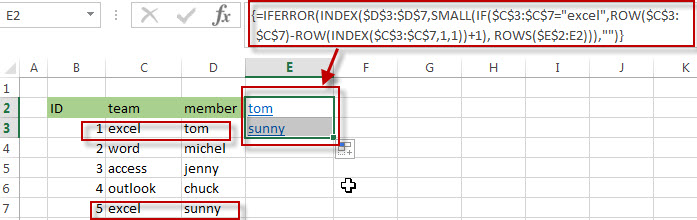If you want to extract the second matches, then put the result into the cell E3, you just need to drag the Fill Handler in Cell E2 to E3. The second matches of the member name should be fetched in the Cell E3.

### Related Formulas

• Get the Position of the nth Occurrence of a Character in a Cell
If you want to get the position of the nth occurrence of a character using a excel formula, you can use the FIND function in combination with the SUBSTITUTE function.
•  Get the position of Last Occurrence of a character or string in a cell
If you want to get the position of the last occurrence of a character in a cell, then you can use a combination of the LOOKUP function, the MID function, the ROW function, the INDIRECT function and the LEN function to create an excel formula.…
• Find the Relative Position in a Range or Table
If you want to know the relative row position for all rows in an Excel Range (B3:D6), you can use a excel Array formula as follows:=ROW(B3:D6)- ROW(B3) + 1. You can also use another excel array formula to get the same result as follows:=ROW(B3:D6)-ROW(INDEX(B3:D6,1,1))+1…
• Get the First Row Number in a Range
If the ROW function use a Range as its argument, it only returns the first row number.You can also use the ROW function within the MIN function to get the first row number in a range. You can also use the INDEX function to get the reference of the first row in a range, then combined to the ROW function to get the first row number of a range.…
•  Get the Last Row Number in a Range
If you want to get the last row number in a range, you need to know the first row number and the total rows number of a range, then perform the addition operation, then subtract 1, the last result is the last row number for that range.…
• Get the position of Last Occurrence of a value in a column
If you want to find the position number of the last occurrence of a specific value in a column (a single range), you can use an array formula with a combination of the MAX function, IF function, ROW function and INDEX Function.

### Related Functions

• Excel IFERROR function
The Excel IFERROR function returns an alternate value you specify if a formula results in an error, or returns the result of the formula.The syntax of the IFERROR function is as below:= IFERROR (value, value_if_error)…
• Excel SMALL function
The Excel SMALL function returns the smallest numeric value from the numbers that you provided. Or returns the smallest value in the array.The syntax of the SMALL function is as below:=SMALL(array,nth) …
• Excel INDEX function
The Excel INDEX function returns a value from a table based on the index (row number and column number)The INDEX function is a build-in function in Microsoft Excel and it is categorized as a Lookup and Reference Function.The syntax of the INDEX function is as below:= INDEX (array, row_num,[column_num])…
• Excel ROW function
The Excel ROW function returns the row number of a cell reference.The ROW function is a build-in function in Microsoft Excel and it is categorized as a Lookup and Reference Function.The syntax of the ROW function is as below:= ROW ([reference])….
• Excel MIN function
The Excel MIN function returns the smallest numeric value from the numbers that you provided. Or returns the smallest value in the array.The MIN function is a build-in function in Microsoft Excel and it is categorized as a Statistical Function.The syntax of the MIN function is as below:= MIN(num1,[num2,…numn])….
• Excel IF function
The Excel IF function perform a logical test to return one value if the condition is TRUE and return another value if the condition is FALSE. The IF function is a build-in function in Microsoft Excel and it is categorized as a Logical Function.The syntax of the IF function is as below:= IF (condition, [true_value], [false_value])….
• Excel ROWS function
The Excel ROWS function returns the number of rows in a cell reference.The ROWS function is a build-in function in Microsoft Excel and it is categorized as a Lookup and Reference Function.The syntax of the ROWS function is as below:= ROWS(array)….
• Excel COLUMNS function
The Excel COLUMNS function returns the number of columns in an Array or a reference.The syntax of the COLUMNS function is as below:=COLUMNS (array)….

## Three Ways to Get the Last Row Number in a Range

This post explains that how to get the last row number in a range or a table in excel. Sometimes, you want to know the last row number for a excel range. The below will guide you how to get the last row number in range using excel formula through the following three ways:

## Get the Last Row Number using ROW and ROWS Functions

If you want to get the last row number in a range, you need to know the first row number and the total rows number of a range, then perform the addition operation, then subtract 1, the last result is the last row number for that range.

We have talked that how to get the first row number in the previous post. And how to get the total row numbers in a range? And you can use the ROWS function. So we can write down the following formula to get the last row number in a range (assuming that B3:D6):

`=ROW(B3:D6) + ROWS(B3:D6)-1`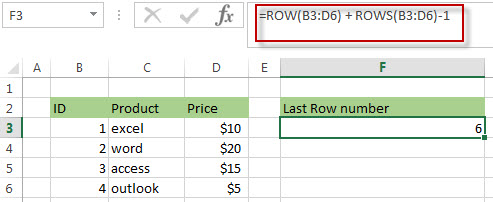The above ROW function returns the first row number in range B3:D6.

=ROWS(B3:D6)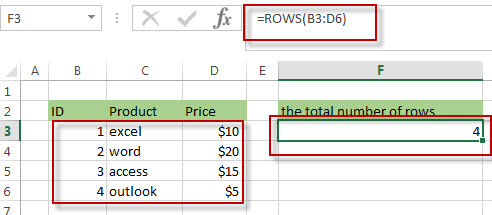The ROWS function returns the total number of rows in range B3:D6.

## Get the Last Row Number using MIN, ROW and ROWS Functions

To get the first row number, you can also use the ROW function within MIN Function as follows:

`=MIN(ROW(B3:D6))`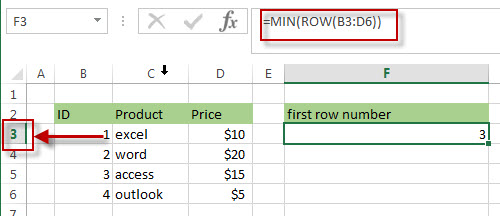So we can use the above formula to create a new formula to get the last row number in a range as follows:

`=MIN(ROW(B3:D6)) + ROWS(B3:D6)-1`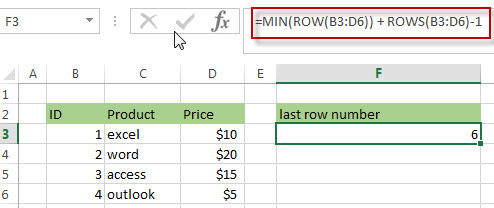## Get the Last Row Number using ROW, INDEX and ROWS Functions

As I said in the previous post, we can use the INDEX function in combination with ROW function to get the first row number in a range. So we can write down another different formula to find the last row number in a range, such as: B3:D6.

`=ROW(INDEX(B3:D6,1,1)) + ROWS(B3:D6)-1`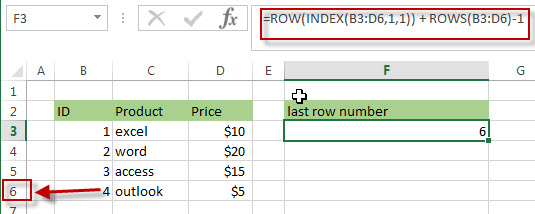### Related Formulas

• Split Text String to an Array
If you want to convert a text string into an array that split each character in text as an element, you can use an excel formula to achieve this result. the below will guide you how to use a combination of the MID function, the ROW function, the INDIRECT function and the LEN function to split a string…
• Find the Relative Position in a Range or Table
If you want to know the relative row position for all rows in an Excel Range (B3:D6), you can use a excel Array formula as follows:=ROW(B3:D6)- ROW(B3) + 1. You can also use another excel array formula to get the same result as follows:=ROW(B3:D6)-ROW(INDEX(B3:D6,1,1))+1…
• Get the First Row Number in a Range
If the ROW function use a Range as its argument, it only returns the first row number.You can also use the ROW function within the MIN function to get the first row number in a range. You can also use the INDEX function to get the reference of the first row in a range, then combined to the ROW function to get the first row number of a range.…

### Related Functions

• Excel INDEX function
The Excel INDEX function returns a value from a table based on the index (row number and column number)The INDEX function is a build-in function in Microsoft Excel and it is categorized as a Lookup and Reference Function.The syntax of the INDEX function is as below:= INDEX (array, row_num,[column_num])…
• Excel ROW function
The Excel ROW function returns the row number of a cell reference.The ROW function is a build-in function in Microsoft Excel and it is categorized as a Lookup and Reference Function.The syntax of the ROW function is as below:= ROW ([reference])….
• Excel ROWS function
The Excel ROWS function returns the number of rows in a cell reference.The ROWS function is a build-in function in Microsoft Excel and it is categorized as a Lookup and Reference Function.The syntax of the ROWS function is as below:=ROWS(array)….
• Excel MIN function
The Excel MIN function returns the smallest numeric value from the numbers that you provided. Or returns the smallest value in the array.The MIN function is a build-in function in Microsoft Excel and it is categorized as a Statistical Function.The syntax of the MIN function is as below:= MIN(num1,[num2,…numn])….

## Excel Rows Function

This post will guide you how to use Excel ROWS function with syntax and examples in Microsoft excel.

## Description

The Excel ROWS function returns the number of rows in a cell reference.

The ROWS function is a build-in function in Microsoft Excel and it is categorized as a Lookup and Reference Function.

The ROWS function is available in Excel 2016, Excel 2013, Excel 2010, Excel 2007, Excel 2003, Excel XP, Excel 2000, Excel 2011 for Mac.

## Syntax

The syntax of the ROWS function is as below:

`= ROWS(array)`

Where the ROWS function arguments is:
array-This is an required argument. An array, or A reference to a range of cells.

## Example

The below examples will show you how to use Excel ROWS Lookup and Reference Function to return the number of rows in a range of cells.

#1 To get the number of rows in the cell range “A1:C4”, just using the following excel formula: =ROWS(A1:C4)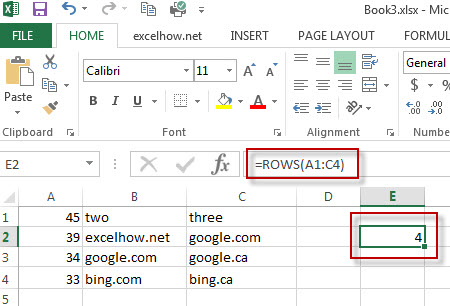### More Excel Rows Examples

• Flip a Column of Data Vertically
How do I remove time part from a date in Excel. How to get only date part from the date with time values via Format cells feature or Excel function. How to extract date from a date and time with VBA code in Excel….
• Randomly Select Cells
If you want to randomly select cells from a range of cells, you can use a formula based on the INDEX function, the RANDBETWEEN function and the Rows function…..
• List all Worksheet Names
Assuming that you have a workbook that has hundreds of worksheets and you want to get a list of all the worksheet names in the current workbook. And the below will introduce 3 methods with you..…
• Split Data in One Column to Multiple Columns
If you want to split each sets in one column from row to row in stacked columns, and you need to create a formula based on the OFFSET function, the COLUMNS function, and the ROWS function..…
• Copy and Paste Only Non-blank Cells
If you want only copy non-blank cells in a range in Excel, you need to select the non-blank cells firstly, then press Ctrl +C keys to copy the selected cells. So how to only select all non-blank cells in the selected range in your worksheet..…